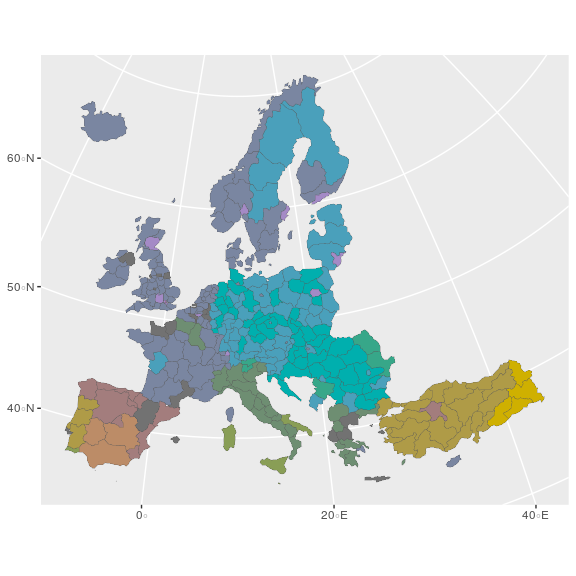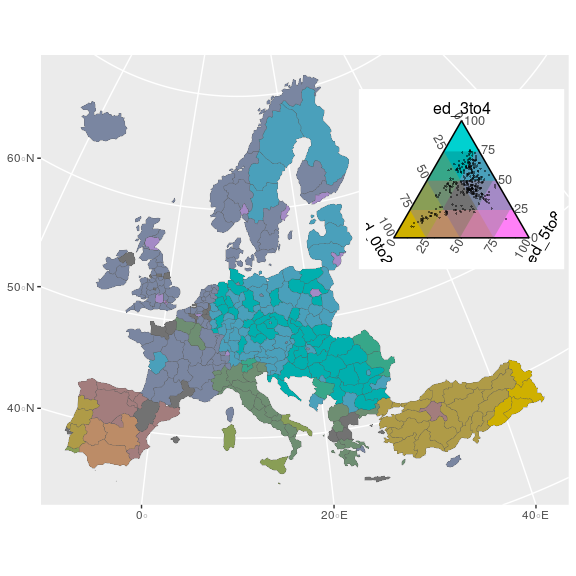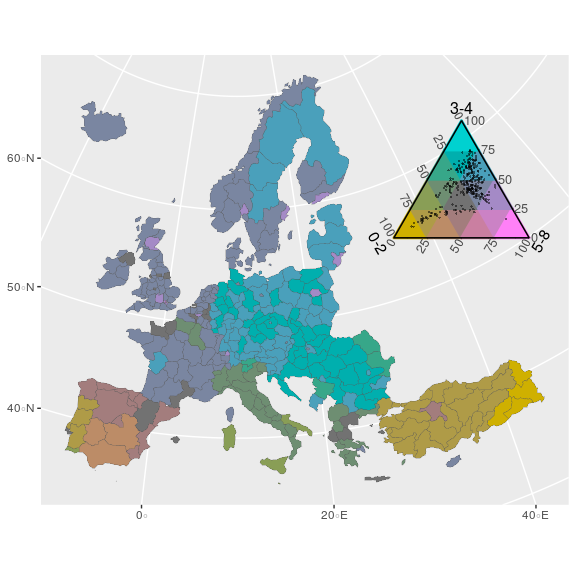# Choropleth maps with tricolore

#### 2020-04-20

Here I demonstrate how to use the `tricolore` library to generate ternary choropleth maps using both `ggplot2` and `leaflet`.

## The data

``````library(tricolore)
#> Registered S3 methods overwritten by 'ggtern':
#>   method           from
#>   grid.draw.ggplot ggplot2
#>   plot.ggplot      ggplot2
#>   print.ggplot     ggplot2``````

The data set `euro_example` contains the administrative boundaries for the European NUTS-2 regions in the column `geometry`. This data can be used to plot a choropleth map of Europe using the `sf` package. Each region is represented by a single row. The name of a region is given by the variable `name` while the respective NUTS-2 geocode is given by the variable `id`. For each region some compositional statistics are available: Variables starting with `ed` refer to the relative share of population ages 25 to 64 by educational attainment in 2016 and variables starting with `lf` refer to the relative share of workers by labor-force sector in the European NUTS-2 regions 2016.

Take the first row of the data set as an example: in the Austrian region of “Burgenland” (`id` = `AT11`) 16.5% of the population aged 25–64 had attained an education of “Lower secondary or less” (`ed_0to2`), 55.7% attained “upper secondary” education (`ed_3to4`), and 27.9% attained “tertiary” education. In the very same region 4.4% of the labor-force works in the primary sector, 26.8% in the secondary and 68.2% in the tertiary sector.

The education and labor-force compositions are ternary, i.e. made up from three elements, and therefore can be color-coded as the weighted mixture of three primary colors, each primary mapped to one of the three elements. Such a color scale is called a ternary balance scheme1. This is what `tricolore` does.

## `ggplot2` for ternary choropleth maps

Here I show how to create a choropleth map of the regional distribution of education attainment in Europe 2016 using `ggplot2`.

1. Using the `Tricolore()` function, color-code each educational composition in the `euro_example` data set and add the resulting vector of hex-srgb colors as a new variable to the data frame. Store the color key seperately.

``````# color-code the data set and generate a color-key
tric <- Tricolore(euro_example, p1 = 'ed_0to2', p2 = 'ed_3to4', p3 = 'ed_5to8')
#> Warning: Ignoring unknown aesthetics: z``````

`tric` contains both a vector of color-coded compositions (`tric\$rgb`) and the corresponding color key (`tric\$key`). We add the vector of colors to the map-data.

``````# add the vector of colors to the `euro_example` data
euro_example\$rgb <- tric\$rgb``````

2. Using `ggplot2` and the joined color-coded education data and geodata, plot a ternary choropleth map of education attainment in the European regions. Add the color key to the map.

The secret ingredient is `scale_fill_identity()` to make sure that each region is colored according to the value in the `rgb` variable of `euro_educ_map`.

``````library(ggplot2)

plot_educ <-
# using sf dataframe `euro_example`...
ggplot(euro_example) +
# ...draw a polygon for each region...
geom_sf(aes(fill = rgb, geometry = geometry), size = 0.1) +
# ...and color each region according to the color code in the variable `rgb`
scale_fill_identity()

plot_educ ``````Using `annotation_custom()` and `ggplotGrob` we can add the color key produced by `Tricolore()` to the map. Internally, the color key is produced with the `ggtern` package. In order for it to render correctly we need to load `ggtern` after loading `ggplot2`. Don’t worry, the `ggplot2` functions still work.

``````library(ggtern)
#> --
#> Remember to cite, run citation(package = 'ggtern') for further info.
#> --
#>
#> Attaching package: 'ggtern'
#> The following objects are masked from 'package:ggplot2':
#>
#>     aes, annotate, ggplot, ggplotGrob, ggplot_build, ggplot_gtable,
#>     ggsave, layer_data, theme_bw, theme_classic, theme_dark,
#>     theme_gray, theme_light, theme_linedraw, theme_minimal, theme_void
plot_educ +
annotation_custom(
ggplotGrob(tric\$key),
xmin = 55e5, xmax = 75e5, ymin = 8e5, ymax = 80e5
)``````Because the color key behaves just like a `ggplot2` plot we can change it to our liking.

``````plot_educ <-
plot_educ +
annotation_custom(
ggplotGrob(tric\$key +
theme(plot.background = element_rect(fill = NA, color = NA)) +
labs(L = '0-2', T = '3-4', R = '5-8')),
xmin = 55e5, xmax = 75e5, ymin = 8e5, ymax = 80e5
)
plot_educ``````Some final touches…

``````plot_educ +
theme_void() +
coord_sf(datum = NA) +
labs(
title = 'European inequalities in educational attainment',
subtitle = 'Regional distribution of ISCED education levels for people aged 25-64 in 2016.'
)``````